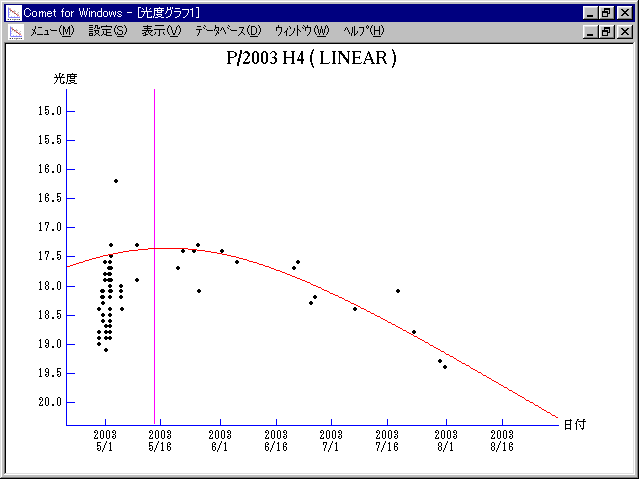# \$B%j%K%"WB@1(B

218P/LINEAR (2003)###\$B%W%m%U%#!<%k(B

 \$BId9f(B 218P/2003 H4 \$BH/8+F|(B 2003\$BG/(B4\$B7n(B29\$BF|(B \$BH/8+8wEY(B 19.0\$BEy(B \$BH/8+ Lincoln Laboratory Near-Earth Asteroid Research project

###\$B###\$B50F;MWAG(B

```                    Epoch = 2003 May   1.0 TT
T = 2003 May  14.30523 TT        Peri. =  10.44779
e = 0.4899747                    Node  = 226.79339 2000.0
q = 1.7030954 AU                 Incl. =  18.14776
a =  3.3392369 AU   n = 0.16152257   P =   6.10 years
```

###\$B@1?^(B###\$B8wEYJQ2=(B

```        m1 = 13.5 + 5 log\$B&\$(B + 20.0 log r
```##### \$B50F;MWAG\$O!"(BCBET 1767\$B\$K7G:\\$5\$l\$?\$b\$N\$G\$9!#(B \$B@1?^\$O(B StellaNavigator Ver.2.0 for Windows (\$B%"%9%H%m%"!<%D(B\$BJTCx(B / \$B%"%9%-!<=PHG6I4)(B) \$B\$G:n@.\$7\$?\$b\$N\$G\$9!#(B \$B8wEY%0%i%U\$O(BComet for Windows\$B\$G:n@.\$7\$?\$b\$N\$G\$9!#(B